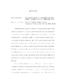## ROBUSTNESS OF ATTRACTING ORBITS2014
##### Authors
Understanding the transition to turbulence is a long-lasting problem in fluid dynamics, particularly in the case of simple flows in which the base laminar flow does not become linearly unstable. For flows at a low Reynolds number, all initial conditions decay to the laminar profile. At higher Reynolds numbers, above a critical value, turbulence is observed, often in the form of a chaotic saddle. The magnitude of the perturbation that disrupts the laminar flow into the turbulent region depends on the Reynolds number and on the direction of the perturbation. In Chapter 2, we investigate the robustness of the laminar attractor to perturbations in a 9-dimensional sinusoidal shear flow model. We examine the geometry of the edge of chaos', where the edge denotes the boundary of the chaotic saddle, which is embedded in the basin of attraction of the laminar state, and is accessible from that state. For a smooth dynamical system $x_{n+1} = F(C, x_n)$ (depending on a parameter C), there may be infinitely many periodic windows, that is, intervals in C having a region of stable periodic behavior. However, the smaller of these windows are easily destroyed with tiny perturbations, so that only finitely many of the windows can be detected for a given level of noise. For a fixed perturbation size $\epsilon$, we consider the system behavior in the presence of noise. In this Chapter, we look at the `$\epsilon$-robust windows'', that is, those periodic windows such that for the superstable parameter value C in that window, the general periodic behavior persists despite noise of amplitude $\le \epsilon$. We focus on the quadratic map, and numerically compute the number of periodic windows that are $\epsilon$-robust. In Chapter 3, we obtain a robustness-exponent $\alpha \approx .51 \pm .03$, which characterizes the robustness of periodic windows in the presence of noise. The character of the time-asymptotic evolution of physical systems can have complex, singular behavior with variation of a system parameter, particularly when chaos is involved. A perturbation of the parameter by a small amount $\epsilon$ can convert an attractor from chaotic to non-chaotic or vice-versa. We call a parameter value where this can happen $\epsilon$-uncertain. The probability that a random choice of the parameter is $\epsilon$-uncertain commonly scales like a power law in $\epsilon$. Surprisingly, two seemingly similar ways of defining this scaling, both of physical interest, yield different numerical values for the scaling exponent. In Chapter 4, we show why this happens and present a quantitative analysis of this phenomenon. Many dynamical systems reach a level of maximum topological entropy as the system parameter is increased followed by a decrease to zero entropy. In Chapter 5, we give an example such that the number of cascades continues to increase for arbitrarily large values of the parameter. We investigate the map $S_{\mu}:[0,1] \rightarrow [0,1)$ defined by $S_{\mu}(x) := \mu \sin(2\pi x) \bmod{1}.$ For this map, the entropy increases without bound as $\mu \rightarrow \infty$, and the system has an ever-increasing number of solitary cascades for $\mu \in [0,m]$ as $m$ is increased to higher and higher integer values. Specifically, we calculate the number of period-$k$ cascades of the map, for $k>1$, for positive integer values of $\mu \in [0,m]$, where $m \in \mathbb{N}$.